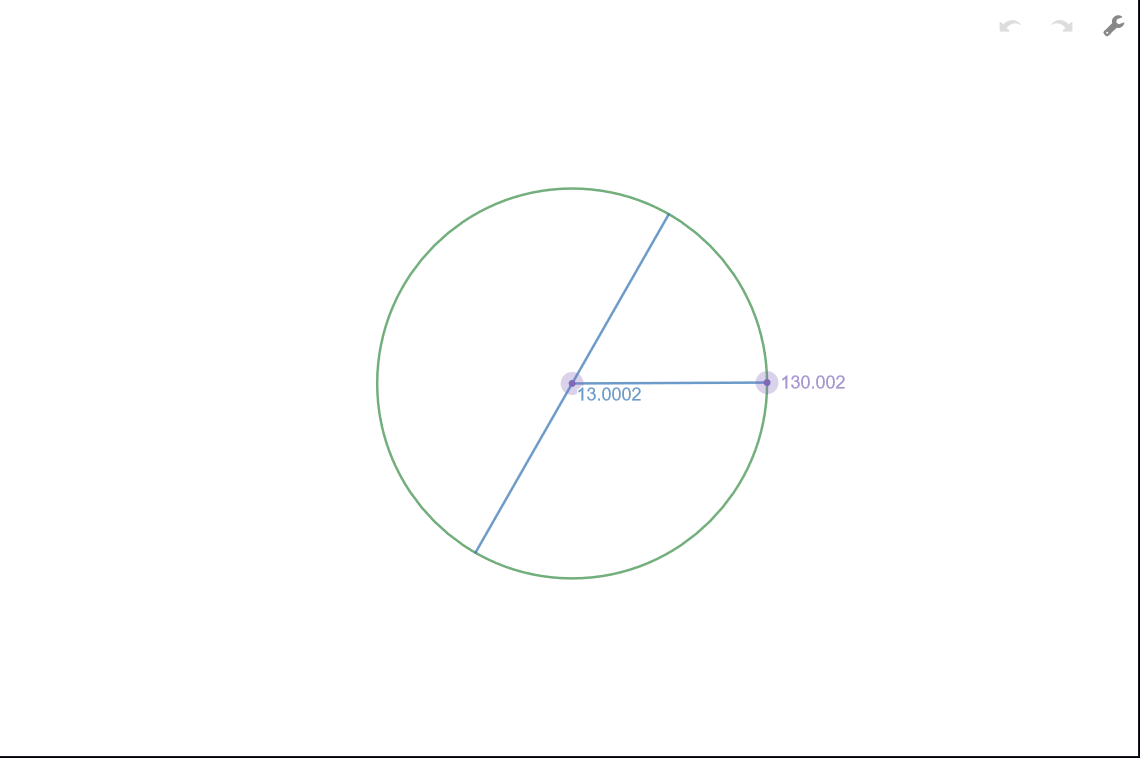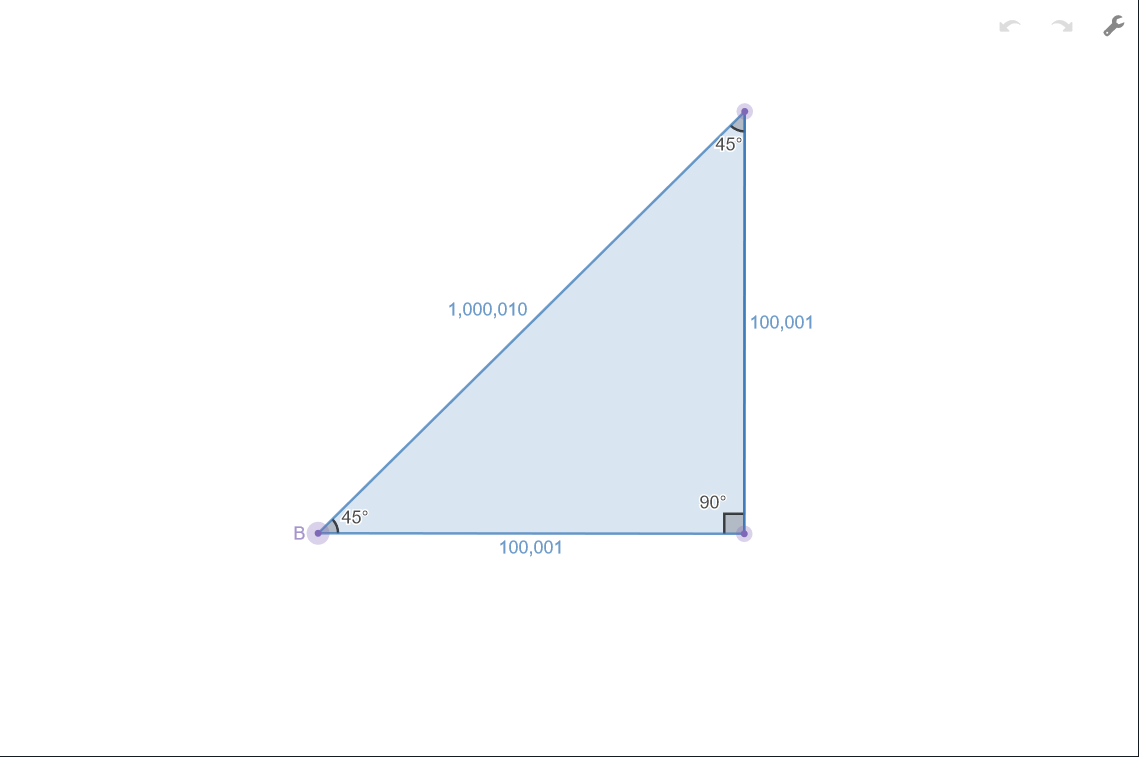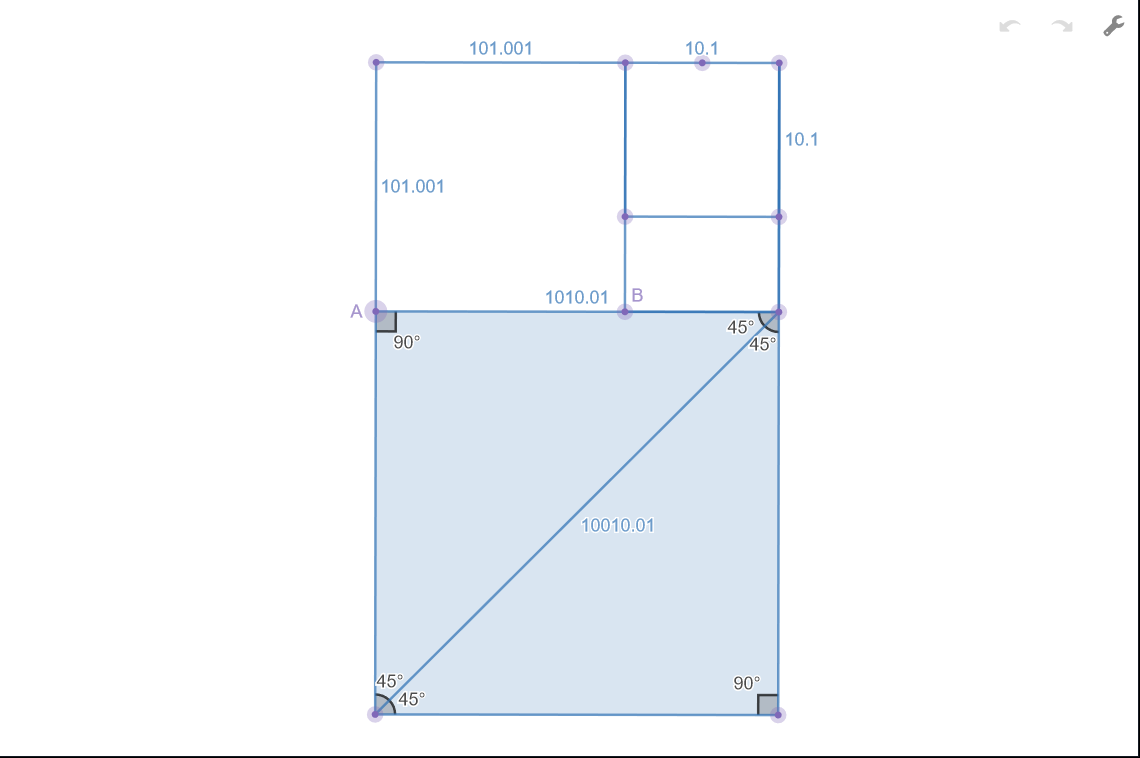Docs
Getting Started
Convert any Number to any Number System.

DecimalSystem is a powerful base conversion library that can convert any (real) number to any base (over 1), including non-integer bases like base PI.

```import {Num} from "decimalsystem";
new Num(10).toBase(Math.PI).toString();```

Demos

The below demos (powered by the Desmos API) use DecimalSystem to help visualize the relationship between some common objects in geometry and their associated constants.

CircleView the relationship between a circle's circumference in base PI.
TriangleView the relationship between the legs and hypotenuse of a 45 degree right triangle in base sqrt(2).
Golden RatioView the relationship between the sides of a golden spiral in base golden ratio.